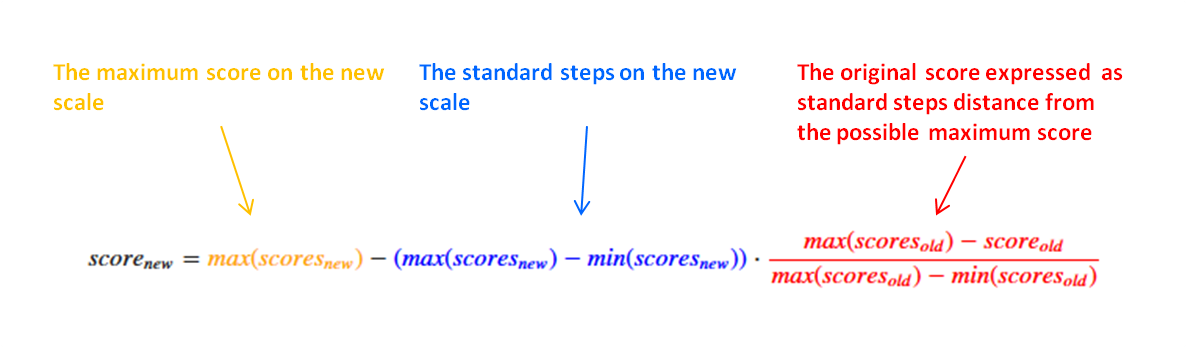# Transforming from one scale to another

## 90 lines of code (Python)

#### Transforming data from one scale to another is such a common task as a data scientist. This blog post goes beyond the options found in sklearn. I have always missed one particular scaler, so in this blog post I write it myself, the ScoreScaler.## Combining data despite different scales

It is very common to compare product score or ratings. A typical example would be a survey which asked for ratings on different scales:

Question Scale Score
How satisfied are you with the product? Between 1 and 5 stars 3 stars
How likely are to recommend the product? Percantage number between 0 and 100 70%
Overall, what grade would you give the customer service? Grade between 1 (best) and 6 (worst) 2

There is a vague feeling that the scores to all three questions are similar. However, when presenting the data you don’t want to burden your audience with mentally translating ratings from one grade to another. How can you bring data to a common scale?

You use a scaler. The Python module `sklearn` offers a range of different scalers (see examples here). However, they all scale according to the observed data. Theoretical minima and maxima of different scales are not taken into account. In surveys, like the example above, very low scores are often not observed, making the scale seem narrower than it really is.

## A new scaler: `score_scale_fun()`

In order to scale according to the full scales, I wrote a new scaler which follows this formula:Note that the min() and max() in this case does not refer to the observed minima and maxima but instead to the theoretically possible minima and maxima on the respective scales. Translated into Python code this would look like this:

``````def score_scale_fun(X, scores_old_min, scores_old_max, scores_new_min=0, scores_new_max=1):
X = scores_new_max - ((scores_new_max - scores_new_min) * (scores_old_max - X) / (scores_old_max - scores_old_min))
return X
``````

Let’s translate the scores of our example to a common scale from zero to five stars using this function. Note that the German grading scale is reversed, meaning that the worst possible score (6) should be seen as the minimum.

``````print(score_scale_fun(3, scores_old_min=1, scores_old_max=5, scores_new_min=0, scores_new_max=5))
print(score_scale_fun(70, scores_old_min=0, scores_old_max=
100, scores_new_min=0, scores_new_max=5))
print(score_scale_fun(2, scores_old_min=6, scores_old_max=1, scores_new_min=0, scores_new_max=5))
``````

The results are now directly comparable:

Question Transformed Scale Score
How satisfied are you with the product? Between 0 and 5 stars 2.5 stars
How likely are to recommend the product? Between 0 and 5 stars 3.5 stars
Overall, what grade would you give the customer service? Between 0 and 5 stars 4.0 stars

Suddenly we see that the customer service was rated much better than the product. This was not obvious prior to rescaling.

## Following the `sklearn` API

Note that `score_scale_fun()` accepts array inputs like `numpy` arrays or `pandas` DataFrames. However, it cannot be used as part of a `sklearn` pipeline. For that the function has to be rewritten as a `sklearn` transformer.

``````from sklearn.base import BaseEstimator
from sklearn.base import TransformerMixin
class ScoreScaler(BaseEstimator, TransformerMixin):
"""Transforms features by scaling each feature to given scoring scale.

This estimator scales and translates each feature individually such
that it acccords with a given range on the training set, e.g. between
zero and one. Without scale arguments, ScoreScaler acts like MinMaxScaler.

Parameters
----------
scores_old_min : int, float, or 'auto'; default 'auto'
The smallest/worst score on the original scale. If 'auto', the smallest value of
each feature is assumed to be the smallest possible value.

scores_old_max : int, float, or 'auto'; default 'auto'
The highest/best score on the original scale. If 'auto', the greatest value of
each feature is assumed to be the highest possible value.

scores_new_min : int or float; default 0
The smallest/worst score on the transformed scale.

scores_new_max : int or float; default 1
The highest/best score on the transformed scale.

Notes
-----
NaNs are treated as missing values: disregarded in fit, and maintained in
transform.
"""

def __init__(self, scores_old_min='auto', scores_old_max='auto', scores_new_min=0, scores_new_max=1):
self.scores_old_min = scores_old_min
self.scores_old_max = scores_old_max
self.scores_new_min = scores_new_min
self.scores_new_max = scores_new_max

def fit(self, X, y=None):
"""Compute the minimum and maximum to be used for later scaling, if no score range is given.

Parameters
----------
X : array-like, shape [n_samples, n_features]
The data used to compute the per-feature minimum and maximum
used for later scaling along the features axis.
"""

if self.scores_old_min == 'auto':
self.scores_old_min_ = X.min()
else:
self.scores_old_min_ = self.scores_old_min

if self.scores_old_max == 'auto':
self.scores_old_max_ = X.max()
else:
self.scores_old_max_ = self.scores_old_max
return self

def transform(self, X):
"""Scaling features of X according to scale settings.

Parameters
----------
X : array-like, shape [n_samples, n_features]
Input data that will be transformed.
"""

X = self.scores_new_max - ((self.scores_new_max - self.scores_new_min) *
(self.scores_old_max_ - X) / (self.scores_old_max_ - self.scores_old_min_))
return X

def inverse_transform(self, X):
"""Undo the scaling of X according to scale settings.

Parameters
----------
X : array-like, shape [n_samples, n_features]
Input data that will be transformed.
"""

X = self.scores_old_max_ - ((self.scores_old_max_ - self.scores_old_min_) *
(self.scores_new_max - X) / (self.scores_new_max - self.scores_new_min))
return X
``````

Such a transformer requires at first an initialisation followed by the use of the object’s `scaler.fit_transform()` method.

``````scaler = ScoreScaler(scores_old_min=1, scores_old_max=5, scores_new_min=0, scores_new_max=5)
scaler.fit_transform(X)
``````

In case, the old scale’s minima and maxima are not available, the `ScoreScaler` just uses the minima and maxima observed in the data.

## Caution

Using the `ScoreScaler` assumes that the data are on different scales but follow the same distribution. When it comes to different traditions of school grades this assumption is wrong (see previous blog bost).

Still, if you have no idea about the distribution of the data and need to transform it, as in the case of a small survey, then the `ScoreScaler` comes in handy. I for one have used it many times.

The complete code to recreate the analyses and plots of this blog post can, as always, be found on github here.